# 3 Linear Dispersion Relation

To obtained the linear dispersion relation, we substitute linearized forms of Eqs. (10)-(15) to the Poisson's equation (16) and restrict up to the first order, which yield: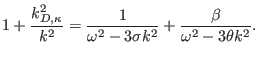(17)

where the appearance of a normalized-dependent screening factor (scaled Debye wavenumber)is defined by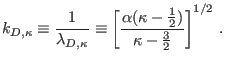(18)

Eq. (17) contains a suprathermality term, a Langmuir wave mode, and a positron wave mode. In this equation,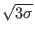corresponds to the normalized cool electron thermal velocity, andis associated with the normalized positron thermal velocity. In the absence of the hot electrons, a linear dispersion is derived in agreement with Eq. 4 im Ref.  and Eq. 8 im Ref. . It is seen that the phase speed increases with higher cool-to-hot electron temperature ratio, in agreement with what found previously . In the limit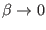(in the absence of the positrons), we obtain Eq. (14) of . From Eq. (17), we see that the frequency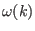, and hence the phase speed, increases with higher positron-to-hot electron temperature ratio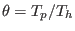. However, this linear thermal effect may not be noticeable due to the range of parameters adopted here, i.e., low values of the positron-to-cool electron density ratio (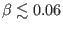).In Figure 1, we plot the dispersion curve (17) in the electron cold limit (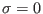), showing the effect of varying the values of the positron-to-cool electron charge density ratio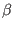. It can be seen that an increase in the positron parameterweakly increases the phase speed (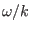). Noting that the positron fraction in astrophysical plasmas, for example in the solar wind , is very small, its effect on the small-amplitude wave solutions is negligible. Otherwise, a large fraction of the positron fraction can significantly modify the dispersion relation. Previously, we found that the dispersion relation and the condition for Landau damping are considerably changed, when the plasma is dominated by hot-distributed electrons (see Figure 1 in Ref. ). Similarly, increasing the number density of suprathermal hot electrons or/and the suprathermality (decreasing) also decreases the phase speed.

Ashkbiz Danehkar
2018-03-28